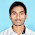Largest of two Numbers without If-Else

This is a simple  Program in C++ for the Largest of 2 without If else statement. This is a part of Mumbai University MCA Colleges Cpp. It is also usually asked in Interviews.

Largest number without If-Else

#include <iostream.h>
int main()
{
float a=3,b=2;

float c=((a+b)/2) + ((a-b)/2);           //c=2.5+0.5=3.0

cout<<"The largest number of the two is "<<c;
return 0;
}

Here we use a simple logic to find the largest of two without using the If Else Statement.

Hope this Program is useful to you in some sense or other. Keep on following this blog for more Mumbai University MCA College Programs. Happy Programming and Studying.

1 comment:

1.very easy program sir..Thank you